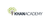# Class 11 Physics (India): Worked example: motion problems (with definite integrals)

Type product
Tijdsduur

## Class 11 Physics (India): Worked example: motion problems (with definite integrals)Khan Academy

Tip: laat jezelf inspireren, bekijk hier de video!### Beschrijving

What can you say about the velocity and position of a particle given its acceleration.

## Class 11 Physics (India)

Let's learn, practice, and master topics of class 11 physics (NCERT) starting with kinematics and then moving to dynamics with Newton's laws of motion, work, energy, and power. Let's then use these as the foundation to learn about centre of mass, rotational motion, gravitation, solids, fluids, thermodynamics, and oscillations and waves.

## Rectilinear motion (integral calc)

Solve problems about motion along a line using the power of integral calculus. For example, given the velocity of a particle as a function of time v(t), find how much the particle has traveled over a given time per…

Lees de volledige beschrijving

### Veelgestelde vragen

Er zijn nog geen veelgestelde vragen over dit product. Als je een vraag hebt, neem dan contact op met onze klantenservice.

Nog niet gevonden wat je zocht? Bekijk deze onderwerpen: Natuurkunde, Biologie, Chemie, Wildbeheer en Zakelijk tekenen.

What can you say about the velocity and position of a particle given its acceleration.

## Class 11 Physics (India)

Let's learn, practice, and master topics of class 11 physics (NCERT) starting with kinematics and then moving to dynamics with Newton's laws of motion, work, energy, and power. Let's then use these as the foundation to learn about centre of mass, rotational motion, gravitation, solids, fluids, thermodynamics, and oscillations and waves.

## Rectilinear motion (integral calc)

Solve problems about motion along a line using the power of integral calculus. For example, given the velocity of a particle as a function of time v(t), find how much the particle has traveled over a given time period.

## Topic: Science

Learn about all the sciences, from physics, chemistry and biology, to cosmology and astronomy, across hundreds of videos, articles and practice questions.

Er zijn nog geen ervaringen.
• Bekijk gerelateerde producten mét ervaringen: Natuurkunde.

### Deel je ervaring

Heb je ervaring met deze cursus? Deel je ervaring en help anderen kiezen. Als dank voor de moeite doneert Springest € 1,- aan Stichting Edukans.

Er zijn nog geen veelgestelde vragen over dit product. Als je een vraag hebt, neem dan contact op met onze klantenservice.